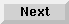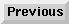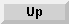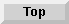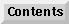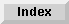2.5 Variable initializations

# 2.5.1 Sequential and parallel initialization

By default, the`with` construct uses sequential initialization of variables; that is, one variable is assigned a value before the next expression is evaluated. However, by using the loop keyword`and` to join several`with` clauses, you can force parallel initialization; that is, all of the specified expressions are evaluated, and the results are bound to the respective variables simultaneously.

Use sequential binding when you want the initialization of some variables to depend on the values of previously bound variables. For example, suppose you want to bind the variables`a`,`b`, and`c` in sequence:

```> (loop with a = 1
with b = (+ a 2)
with c = (+ b 3)
return (list a b c))
(1 3 6)

```
The execution of the above loop is equivalent to the execution of the following code:

```(let* ((a 1)
(b (+ a 2))
(c (+ b 3)))
(block nil
(tagbody
next-loop (return (list a b c))
(go next-loop)
end-loop)))

```
If you are not depending on the value of previously bound variables for the initialization of other local variables, you can use an`and` clause to force the bindings to occur in parallel:

```> (loop with a = 1
and b = 2
and c = 3
return (list a b c))
(1 2 3)

```
The execution of the above loop is equivalent to the execution of the following code:

```(let ((a 1)
(b 2)
(c 3))
(block nil
(tagbody
next-loop (return (list a b c))
(go next-loop)
end-loop)))

```

The Loop Facility - 9 SEP 1996Generated with Harlequin WebMaker